# Variants of Solomonoff induction

This page lists some variants of Solomonoff induction. Solomonoff induction is an idealized method of sequence prediction

For determinism, I think "deterministic" is the same as "Solomonoff prior" and "stochastic" is the same as "universal mixture". Sterkenburg calls deterministic versions a "bottom-up approach" whereas the universal mixture is a "top-down approach" (p. 30). For deterministic variants, the type of universal machine must be specified. With universal mixtures, one must specify two things: the weighting to use, and the class of distributions to consider.

For discrete vs continuous, I think this just means whether the prior we define is over finite strings or over infinite sequences (where we want to know the probability of an infinite sequence starting with a given finite string). I'm not sure how to tell whether a given formula is discrete or continuous. One difference seems to be that with discrete semimeasures, we only require that the sum is at most 1, whereas with continuous semimeasures we also require that$\mu(x) \geq \mu(x0) + \mu(x1)$? (see e.g. p. 5 of  and p. 294 of Li and Vitanyi) Apparently one way to think of the discrete version is to think of the sample space as the natural numbers, i.e. one-letter strings from a countably infinite alphabet (see p. 265 of Li and Vitanyi).

NOTE: as of july 2019 i'm no longer sure that discrete vs continuous is actually a distinction. i think all(?) versions of solomonoff induction use continuous semimeasures. i'm not sure why the discrete one can't be used though. e.g. "For applications such as the prediction of growing sequences it is necessary to define a similar distribution on infinite binary sequences"  Solomonoff also says similar things in at least one of his papers. February 2020 update: there is a discrete vs continuous distinction after all. With a discrete semimeasure,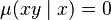$\mu(xy\mid x) = 0$ for all non-empty strings y, since we are considering ordinary Turing machines that halt. if a TM halts with output x, then it can't also halt with output xy. So the universal discrete semimeasure can't be used for sequence prediction, since all conditional probabilities are zero, hence useless. To do sequence prediction, we need to switch to monotone TMs and allow partial outputs.

Source Formula Determinism Discrete vs continuous Notes
LessWrong Wiki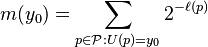$m(y_0) = \sum_{p \in \mathcal P : U(p) = y_0} 2^{-\ell(p)}$ where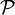$\mathcal P$ is the set of self-delimiting programs Deterministic; prefix Turing machine Discrete
Scholarpedia discrete universal a priori probability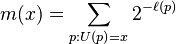$m(x) = \sum_{p:U(p)=x} 2^{-\ell(p)}$ where the sum is over halting (i.e. self-delimiting) programs Deterministic; prefix Turing machine Discrete In this notation,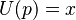$U(p) = x$ means that$U$, when run with program$p$, halts having just read all of$p$ (and not beyond$p$). This means that "halting program" is the same thing as "self-delimiting program".
Scholarpedia continuous universal a priori probability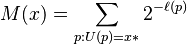$M(x) = \sum_{p : U(p) = x*} 2^{-\ell(p)}$ where the sum is over minimal programs Deterministic; monotone Turing machine Continuous
Sterkenburg (p. 22)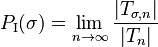$P_{\mathrm{I}}(\sigma) = \lim_{n\to\infty} \frac{|T_{\sigma,n}|}{|T_n|}$ where$\sigma$ is a finite string,$T_n$ is the set of all halting (valid) inputs of length$n$ to the reference machine$U$,$T_{\sigma,n}$ is the set of all halting (valid) inputs of length$n$ that output something starting with$\sigma$ Deterministic; ordinary Turing machine Discrete (I think because we are only considering halting inputs, the sample space is the set of all finite strings, which is a countable/discrete set) this seems similar to solomonoff's section 3.3. also I think this is different from the scholarpedia$m(x)$ because here we allow anything that outputs starting with x to count toward the probability, rather than exactly x.
Sterkenburg (p. 24)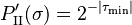$P'_{\mathrm{II}}(\sigma) = 2^{-|\tau_\mathrm{min}|}$ where$\tau_\mathrm{min}$ is the shortest program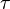$\tau$ such that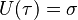$U(\tau) = \sigma$ (i.e. the shortest program that causes the reference machine to output$\sigma$ and halt) deterministic; universal Turing machine, universal prefix machine (to get a probability distribution; see remark on p. 27) discrete? this formula does not define a probability distribution over strings$\sigma$ because the sum of probabilities does not converge
Sterkenburg (p. 25)$P''_{\mathrm{II}}(\sigma) = \lim_{n\to\infty} \sum_{\tau \in T_{\sigma,n}} 2^{-|\tau|}$ where$T_{\sigma,n}$ is the set of all programs$\tau$ of length$n$ such that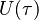$U(\tau)$ begins with$\sigma$ deterministic; universal Turing machine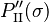$P''_{\mathrm{II}}(\sigma)$ is divergent even for a single$\sigma$, so this is not actually a workable version, but is intended as a stepping stone
Sterkenburg (p. 26)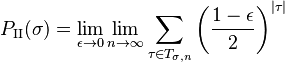$P_{\mathrm{II}}(\sigma) = \lim_{\epsilon\to 0} \lim_{n\to \infty} \sum_{\tau \in T_{\sigma,n}} \left(\frac{1-\epsilon}{2}\right)^{|\tau|}$ deterministic; universal Turing machine, universal prefix machine (to get a probability distribution; see remark on p. 27) The use of the$\epsilon$ is a hack to get the sum to converge
Sterkenburg (p. 29)$Q_U(\sigma) = \sum_{\tau \in T_\sigma} 2^{-|\tau|}$ where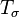$T_\sigma$ is the set of minimal descriptions of$\sigma$ (i.e. set of programs that output something starting with$\sigma$ such that if one removes one bit from the end of the program, it no longer outputs something starting with$\sigma$) deterministic; universal monotone machine continuous?
Sterkenburg (p. 31)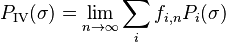$P_{\mathrm{IV}}(\sigma) = \lim_{n\to\infty} \sum_i f_{i,n}P_i(\sigma)$ Stochastic; I think this is the same as Solomonoff's section 3.4, eq. 13, except that Solomonoff takes the limit of the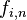$f_{i,n}$ separately and then plugs it into the formula, rather than taking the limit of the sum.
Sterkenburg (p. 33)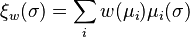$\xi_w(\sigma) = \sum_i w(\mu_i)\mu_i(\sigma)$ Stochastic; weighting unspecified, except for the requirement that$w(\mu_i)>0$ for all$i$ and that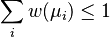$\sum_i w(\mu_i) \leq 1$; the model class is all semicomputable semimeasures
Arbital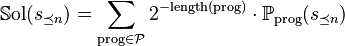$\mathbb{S}\mathrm{ol}(s_{\preceq n}) = \sum_{\mathrm{prog} \in \mathcal{P}} 2^{-\mathrm{length}(\mathrm{prog})} \cdot \mathbb P_{\mathrm{prog}}(s_{\preceq n})$ Stochastic; the weighting is 1/2 to the power of the length of the program; the model class is all programs that define a probability measure (?), where the programs are given using a prefix-free code
Solomonoff section 3.1, eq. 1$\lim_{\epsilon\to0} \lim_{n\to\infty} \sum_{k=1}^{r^n} \sum_{i=1}^\infty ((1-\epsilon)/2)^{N_{(S_{TC_{n,k}})_i}}$ (this might be incorrect). Solomonoff is using the notation$C_{n,k}$ to mean that he doesn't care about what happens as long as the desired string$T$ starts the output. In more modern notation we would say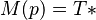$M(p)=T*$ or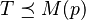$T \preceq M(p)$ rather than$M(p) = TC_{n,k}$ (although note that with Solomonoff's notation, we are also restricting the length of the output, so in the modern notation we would have to also require that$|M(p)| = |T| + n$). Deterministic; universal Turing machine Solomonoff originally gave the conditional probability of seeing$a$ given we've already seen$T$, which he writes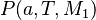$P(a,T,M_1)$ but which in more common notation would be something like$P_{M_1}(Ta\mid T)$.
Solomonoff section 3.2, eq. 7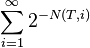$\sum_{i=1}^\infty 2^{-N(T,i)}$ where$N(T,i)$ is the number of bits in the$i$th minimal program for$T$ Deterministic; universal monotone machine
Solomonoff section 3.3, eq. 9 and 11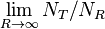$\lim_{R\to\infty} N_T/N_R$, where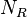$N_R$ is the number of programs of length$R$ that cause the machine to halt eventually, and$N_T$ are the subset of those programs that cause the machine to output something starting with$T$ Deterministic; either a universal Turing machine or a universal monotone machine
Solomonoff section 3.3, eq. 10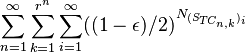$\sum_{n=1}^\infty \sum_{k=1}^{r^n} \sum_{i=1}^\infty ((1-\epsilon)/2)^{N_{(S_{TC_{n,k}})_i}}$ Deterministic; Solomonoff says this follows from equation 10, but I'm not sure how. In more modern notation, I think this can be written as$\sum_{p:U(p)=T*} \left(\frac{1-\epsilon}{2}\right)^{|p|}$
Solomonoff section 3.4, eq. 13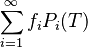$\sum_{i=1}^\infty f_i P_i(T)$ Stochastic i think solomonoff is using all computable measures (rather than enumerable semimeasures)
Legg$\mathbf M(x) = \sum_{\mu \in \mathcal M^R} Q^{-H(\mu)} \mu(x)$ where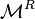$\mathcal M^R$ is the set of all computable semimeasures,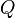$Q$ is the number of symbols in the alphabet, and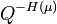$Q^{-H(\mu)}$ is an approximation of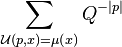$\sum_{\mathcal U(p,x)=\mu(x)} Q^{-|p|}$ Stochastic Legg is summing over all the semimeasures rather than programs that compute semimeasures, which is why he needs to define the weights$Q^{-H(\mu)}$ rather than just taking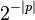$2^{-|p|}$.